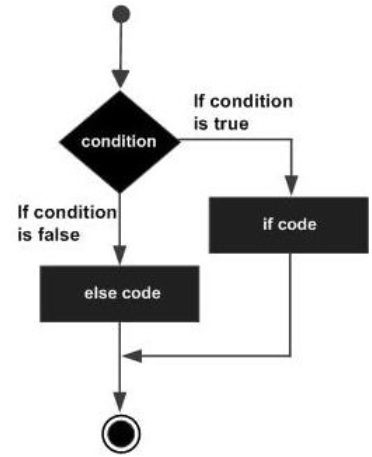# Batch Script - If/else Statement

The next decision making statement is the If/else statement. Following is the general form of this statement.

```If (condition) (do_something) ELSE (do_something_else)
```

The general working of this statement is that first a condition is evaluated in the ‘if’ statement. If the condition is true, it then executes the statements thereafter and stops before the else condition and exits out of the loop. If the condition is false, it then executes the statements in the else statement block and then exits the loop. The following diagram shows the flow of the ‘if’ statement.## Checking Variables

Just like the ‘if’ statement in Batch Script, the if-else can also be used for checking variables which are set in Batch Script itself. The evaluation of the ‘if’ statement can be done for both strings and numbers.

### Checking Integer Variables

The following example shows how the ‘if’ statement can be used for numbers.

Example

```@echo off
SET /A a = 5
SET /A b = 10
SET /A c = %a% + %b%
if %c%==15 (echo "The value of variable c is 15") else (echo "Unknown value")
if %c%==10 (echo "The value of variable c is 10") else (echo "Unknown value")
```

The key thing to note about the above program is −

• Each ‘if else’ code is placed in the brackets (). If the brackets are not placed to separate the code for the ‘if and else’ code, then the statements would not be valid proper if else statements.

• In the first ‘if else’ statement, the if condition would evaluate to true.

• In the second ‘if else’ statement, the else condition will be executed since the criteria would be evaluated to false.

Output

The above command produces the following output.

```"The value of variable c is 15"
"Unknown value"
```

### Checking String Variables

The same example can be repeated for strings. The following example shows how the ‘if else’ statement can be used to strings.

Example

```@echo off
SET str1 = String1
SET str2 = String2

if %str1%==String1 (echo "The value of variable String1") else (echo "Unknown value")
if %str2%==String3 (echo "The value of variable c is String3") else (echo "Unknown value")
```

The key thing to note about the above program is −

• The first ‘if’ statement checks if the value of the variable str1 contains the string “String1”. If so, then it echo’s a string to the command prompt.

• Since the condition of the second ‘if’ statement evaluates to false, the echo part of the statement will not be executed.

Output

The above command produces the following output.

```"The value of variable String1"
"Unknown value"
```

### Checking Command Line Arguments

The ‘if else’ statement can also be used for checking of command line arguments. The following example show how the ‘if’ statement can be used to check for the values of the command line arguments.

Example

```@echo off
echo %1
echo %2
echo %3
if %1%==1 (echo "The value is 1") else (echo "Unknown value")
if %2%==2 (echo "The value is 2") else (echo "Unknown value")
if %3%==3 (echo "The value is 3") else (echo "Unknown value")
```

Output

If the above code is saved in a file called test.bat and the program is executed as

```test.bat 1 2 4
```

Following will be the output of the above program.

```1
2
4
"The value is 1"
"The value is 2"
"Unknown value"
```

## if defined

A special case for the ‘if’ statement is the "if defined", which is used to test for the existence of a variable. Following is the general syntax of the statement.

```if defined somevariable somecommand
```

Following is an example of how the ‘if defined’ statement can be used.

Example

```@echo off
SET str1 = String1
SET str2 = String2
if defined str1 echo "Variable str1 is defined"

if defined str3 (echo "Variable str3 is defined") else (echo "Variable str3 is not defined")
```

Output

The above command produces the following output.

```"Variable str1 is defined"
"Variable str3 is not defined"
```

## if exists

Another special case for the ‘if’ statement is the "if exists ", which is used to test for the existence of a file. Following is the general syntax of the statement.

```If exist somefile.ext do_something
```

Following is an example of how the ‘if exists’ statement can be used.

Example

```@echo off
if exist C:\set2.txt echo "File exists"
if exist C:\set3.txt (echo "File exists") else (echo "File does not exist")
```

Output

Let’s assume that there is a file called set2.txt in the C drive and that there is no file called set3.txt. Then, following will be the output of the above code.

```"File exists"
"File does not exist"
```
batch_script_decision_making.htm